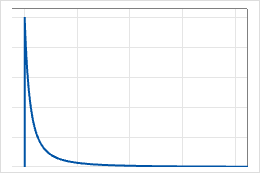# Loglogistic distribution

Use the loglogistic distribution when the logarithm of the variable is logistically distributed. For example, the loglogistic distribution is used in growth models and to model binary responses in fields such as biostatistics and economics.

The loglogistic distribution is a continuous distribution that is defined by its scale and location parameters. The 3-parameter loglogistic distribution is defined by its scale, location, and threshold parameters.

The following graph illustrates the loglogistic distribution for scale=1.0, location=0.0, and threshold=0.0.The loglogistic distribution is also known as the Fisk distribution.

By using this site you agree to the use of cookies for analytics and personalized content.  Read our policy